1594899240

# An Introduction to Gradient Descent and Backpropagation

## How the Machine Learns?

Machine learning (ML) is the study of computer algorithms that improve automatically through experience. It is seen as a subset of _artificial intelligence. Machine learning algorithms build a mathematical model based on sample data, known as “training data”, in order to make predictions or decisions without being explicitly programmed to do so.

We, as humans can study data to find the behavior and predict something based on the behavior, but a machine can’t really operate like us. So, in most cases, it tries to learn from already established examples. Say, for a classic classification problem, we have a lot of examples from which the machine learns. Each example is a particular circumstance or description which is depicted by a combination of features and their corresponding labels. In our real-world, we have a different description for every different object and, we know these different objects by different names. For example, cars and bikes are just two object names or two labels. They have different descriptions like the number of wheels is two for a bike and four for a car. So, the number of wheels can be used to differentiate between a car and a bike. It can be a feature to differentiate between these two labels.

Every common aspect of the description of different objects which can be used to differentiate it from one another is fit to be used as a feature for the unique identification of a particular object among the others. Similarly, we can assume, the age of a house, the number of rooms and the position of the house will play a major role in deciding the costing of a house. This is also very common in the real world. So, these aspects of the description of the house can be really useful for predicting the house price, as a result, they can be really good features for such a problem.

In machine learning, we have mainly two types of problems, classification, and regression. The identification between a car and a bike is an example of a classification problem and the prediction of the house price is a regression problem.

We have seen for any type of problem, we basically depend upon the different features corresponding to an object to reach a conclusion. The machine does a similar thing to learn. It also depends on the different features of objects to reach a conclusion. Now, in order to differentiate between a car and a bike, which feature will you value more, the number of wheels or the maximum speed or the color? The answer is obviously first the number of wheels, then the maximum speed, and then the color. The machine does the same thing to understand which feature to value most, it assigns some weights to each feature, which helps it understand which feature is most important among the given ones.

Now, it tries to devise a formula, like say for a regression problem,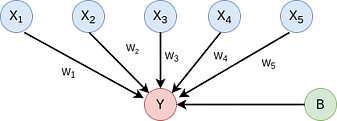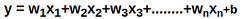Equation 1

Here w1,w2, w3 are the weights of there corresponding features like x1,x2, x3 and b is a constant called the bias. Its importance is that it gives flexibility. So, using such an equation the machine tries to predict a value y which may be a value we need like the price of the house. Now, the machine tries to perfect its prediction by tweaking these weights. It does so, by comparing the predicted value y with the actual value of the example in our training set and using a function of their differences. This function is called a loss function.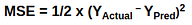Equation 2

The machine tries to decrease this loss function or the error, i.e tries to get the prediction value close to the actual value.

This method is the key to minimizing the loss function and achieving our target, which is to predict close to the original value.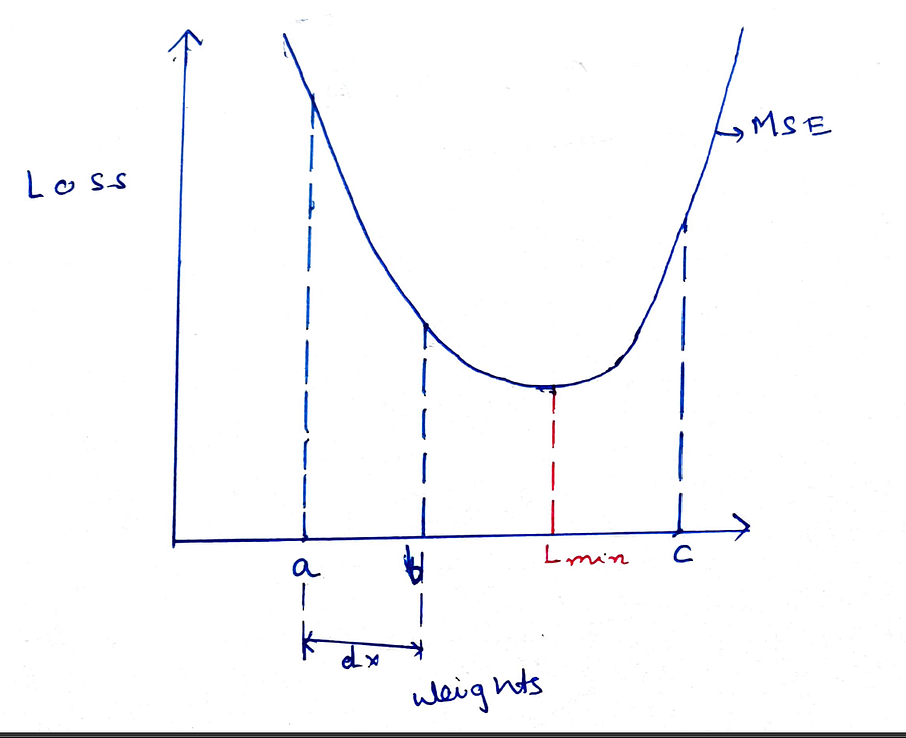In this diagram, above we see our loss function graph. If we observe we will see it is basically a parabolic shape or a convex shape, it has a specific global minimum which we need to find in order to find the minimum loss function value. So, we always try to use a loss function which is convex in shape in order to get a proper minimum. Now, we see the predicted results depend on the weights from the equation. If we replace equation 1 in equation 2 we obtain this graph, with weights in X-axis and Loss on Y-axis.

Initially, the model assigns random weights to the features. So, say it initializes the weight=a. So, we can see it generates a loss which is far from the minimum point L-min.

Now, we can see that if we move the weights more towards the positive x-axis we can optimize the loss function and achieve minimum value. But, how will the machine know? We need to optimize weight to minimize error, so, obviously, we need to check how the error varies with the weights. To do this we need to find the derivative of the Error with respect to the weight. This derivative is called Gradient.

Where E is the error and w is the weight.

Let’s see how this works. Say, if the loss increases with an increase in weight so Gradient will be positive, So we are basically at the point C, where we can see this statement is true. If loss decreases with an increase in weight so gradient will be negative. We can see point A, corresponds to such a situation. Now, from point A we need to move towards positive x-axis and the gradient is negative. From point C, we need to move towards negative x-axis but the gradient is positive. So, always the negative of the Gradient shows the directions along which the weights should be moved in order to optimize the loss function. So, this way the gradient guides the model whether to increase or decrease weights in order to optimize the loss function

## Buddha Community1603753200

## ML Optimization pt.1 - Gradient Descent with Python

So far in our journey through the Machine Learning universe, we covered several big topics. We investigated some regression algorithms, classification algorithms and algorithms that can be used for both types of problems (SVM**, **Decision Trees and Random Forest). Apart from that, we dipped our toes in unsupervised learning, saw how we can use this type of learning for clustering and learned about several clustering techniques.

We also talked about how to quantify machine learning model performance and how to improve it with regularization. In all these articles, we used Python for “from the scratch” implementations and libraries like TensorFlowPytorch and SciKit Learn. The word optimization popped out more than once in these articles, so in this and next article, we focus on optimization techniques which are an important part of the machine learning process.

In general, every machine learning algorithm is composed of three integral parts:

1. loss function.
2. Optimization criteria based on the loss function, like a cost function.
3. Optimization technique – this process leverages training data to find a solution for optimization criteria (cost function).

As you were able to see in previous articles, some algorithms were created intuitively and didn’t have optimization criteria in mind. In fact, mathematical explanations of why and how these algorithms work were done later. Some of these algorithms are Decision Trees and kNN. Other algorithms, which were developed later had this thing in mind beforehand. SVMis one example.

During the training, we change the parameters of our machine learning model to try and minimize the loss function. However, the question of how do you change those parameters arises. Also, by how much should we change them during training and when. To answer all these questions we use optimizers. They put all different parts of the machine learning algorithm together. So far we mentioned Gradient Decent as an optimization technique, but we haven’t explored it in more detail. In this article, we focus on that and we cover the grandfather of all optimization techniques and its variation. Note that these techniques are not machine learning algorithms. They are solvers of minimization problems in which the function to minimize has a gradient in most points of its domain.

### Dataset & Prerequisites

Data that we use in this article is the famous Boston Housing Dataset . This dataset is composed 14 features and contains information collected by the U.S Census Service concerning housing in the area of Boston Mass. It is a small dataset  with only 506 samples.For the purpose of this article, make sure that you have installed the following _Python _libraries:

• **NumPy **– Follow this guide if you need help with installation.
• **SciKit Learn **– Follow this guide if you need help with installation.
• Pandas – Follow this guide if you need help with installation.

Once installed make sure that you have imported all the necessary modules that are used in this tutorial.

``````import pandas as pd
import numpy as np
import matplotlib.pyplot as plt

from sklearn.model_selection import train_test_split
from sklearn.metrics import mean_squared_error
from sklearn.preprocessing import StandardScaler
from sklearn.linear_model import SGDRegressor
``````

Apart from that, it would be good to be at least familiar with the basics of linear algebracalculus and probability.

### Why do we use Optimizers?

Note that we also use simple Linear Regression in all examples. Due to the fact that we explore optimizationtechniques, we picked the easiest machine learning algorithm. You can see more details about Linear regression here. As a quick reminder the formula for linear regression goes like this: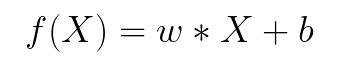where w and b are parameters of the machine learning algorithm. The entire point of the training process is to set the correct values to the w and b, so we get the desired output from the machine learning model. This means that we are trying to make the value of our error vector as small as possible, i.e. to find a global minimum of the cost function.

One way of solving this problem is to use calculus. We could compute derivatives and then use them to find places where is an extrema of the cost function. However, the cost function is not a function of one or a few variables; it is a function of all parameters of a machine learning algorithm, so these calculations will quickly grow into a monster. That is why we use these optimizers.

#ai #machine learning #python #artificaial inteligance #artificial intelligence #batch gradient descent #data science #datascience #deep learning #from scratch #gradient descent #machine learning #machine learning optimizers #ml optimization #optimizers #scikit learn #software #software craft #software craftsmanship #software development #stochastic gradient descent1594899240

## How the Machine Learns?

Machine learning (ML) is the study of computer algorithms that improve automatically through experience. It is seen as a subset of _artificial intelligence. Machine learning algorithms build a mathematical model based on sample data, known as “training data”, in order to make predictions or decisions without being explicitly programmed to do so.

We, as humans can study data to find the behavior and predict something based on the behavior, but a machine can’t really operate like us. So, in most cases, it tries to learn from already established examples. Say, for a classic classification problem, we have a lot of examples from which the machine learns. Each example is a particular circumstance or description which is depicted by a combination of features and their corresponding labels. In our real-world, we have a different description for every different object and, we know these different objects by different names. For example, cars and bikes are just two object names or two labels. They have different descriptions like the number of wheels is two for a bike and four for a car. So, the number of wheels can be used to differentiate between a car and a bike. It can be a feature to differentiate between these two labels.

Every common aspect of the description of different objects which can be used to differentiate it from one another is fit to be used as a feature for the unique identification of a particular object among the others. Similarly, we can assume, the age of a house, the number of rooms and the position of the house will play a major role in deciding the costing of a house. This is also very common in the real world. So, these aspects of the description of the house can be really useful for predicting the house price, as a result, they can be really good features for such a problem.

In machine learning, we have mainly two types of problems, classification, and regression. The identification between a car and a bike is an example of a classification problem and the prediction of the house price is a regression problem.

We have seen for any type of problem, we basically depend upon the different features corresponding to an object to reach a conclusion. The machine does a similar thing to learn. It also depends on the different features of objects to reach a conclusion. Now, in order to differentiate between a car and a bike, which feature will you value more, the number of wheels or the maximum speed or the color? The answer is obviously first the number of wheels, then the maximum speed, and then the color. The machine does the same thing to understand which feature to value most, it assigns some weights to each feature, which helps it understand which feature is most important among the given ones.

Now, it tries to devise a formula, like say for a regression problem,Equation 1

Here w1,w2, w3 are the weights of there corresponding features like x1,x2, x3 and b is a constant called the bias. Its importance is that it gives flexibility. So, using such an equation the machine tries to predict a value y which may be a value we need like the price of the house. Now, the machine tries to perfect its prediction by tweaking these weights. It does so, by comparing the predicted value y with the actual value of the example in our training set and using a function of their differences. This function is called a loss function.Equation 2

The machine tries to decrease this loss function or the error, i.e tries to get the prediction value close to the actual value.

This method is the key to minimizing the loss function and achieving our target, which is to predict close to the original value.In this diagram, above we see our loss function graph. If we observe we will see it is basically a parabolic shape or a convex shape, it has a specific global minimum which we need to find in order to find the minimum loss function value. So, we always try to use a loss function which is convex in shape in order to get a proper minimum. Now, we see the predicted results depend on the weights from the equation. If we replace equation 1 in equation 2 we obtain this graph, with weights in X-axis and Loss on Y-axis.

Initially, the model assigns random weights to the features. So, say it initializes the weight=a. So, we can see it generates a loss which is far from the minimum point L-min.

Now, we can see that if we move the weights more towards the positive x-axis we can optimize the loss function and achieve minimum value. But, how will the machine know? We need to optimize weight to minimize error, so, obviously, we need to check how the error varies with the weights. To do this we need to find the derivative of the Error with respect to the weight. This derivative is called Gradient.

Where E is the error and w is the weight.

Let’s see how this works. Say, if the loss increases with an increase in weight so Gradient will be positive, So we are basically at the point C, where we can see this statement is true. If loss decreases with an increase in weight so gradient will be negative. We can see point A, corresponds to such a situation. Now, from point A we need to move towards positive x-axis and the gradient is negative. From point C, we need to move towards negative x-axis but the gradient is positive. So, always the negative of the Gradient shows the directions along which the weights should be moved in order to optimize the loss function. So, this way the gradient guides the model whether to increase or decrease weights in order to optimize the loss function1625043360

## Understanding Gradient Descent with Python

So far in our journey through the Machine Learning universe, we covered several big topics. We investigated some regression algorithms, classification algorithms and algorithms that can be used for both types of problems (SVM, Decision Trees and Random Forest). Apart from that, we dipped our toes in unsupervised learning, saw how we can use this type of learning for clustering and learned about several clustering techniques.

We also talked about how to quantify machine learning model performance and how to improve it with regularization. In all these articles, we used Python for “from the scratch” implementations and libraries like TensorFlowPytorch and SciKit Learn. The word optimization popped out more than once in these articles, so in this article, we focus on optimization techniques which are an important part of the machine learning process.

#ai #machine learning #python #artificaial inteligance #artificial intelligence #batch gradient descent #data science #datascience #deep learning #from scratch #gradient descent #machine learning optimizers #ml optimization #optimizers #scikit learn #software #software craft #software craftsmanship #software development1599353580

## An Introduction to Gradient Descent

Gradient Descent is an optimization algorithm used to tweak parameters iteratively to minimize the cost function.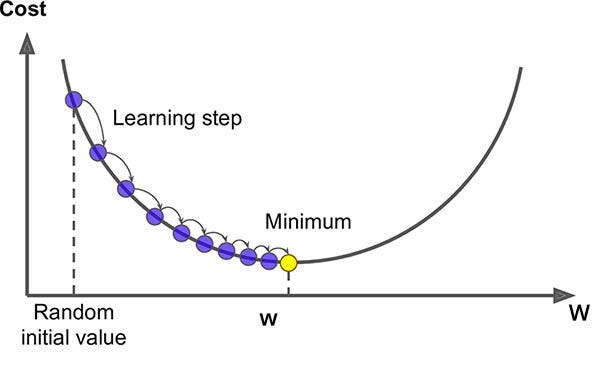Source: Saugat Bhattarai

Suppose you’re blindfolded in the mountains, and your goal is to reach the bottom of the valley swiftly.

There are many solutions to reach your goal. One of the good strategy is to go downhill in the direction of the steepest slope.

This is what Gradient Descent does. It measures the local gradient of the error function with regard to the parameter, and it goes in the direction of descending gradient. It reached a minimum once the gradient is zero.

### Learning Rate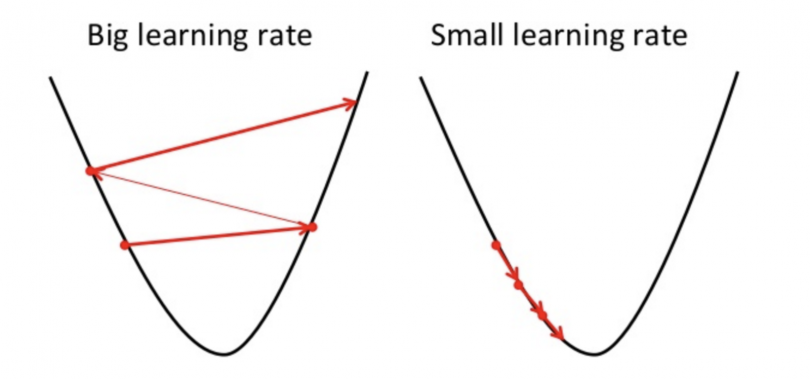Source: Towards Data Science

The learning rate hyperparameter is an important parameter in Gradient Descent, which is the size of the steps.

If the learning rate is too high, the algorithm might jump across the valley and possibly even higher than before. This might result in the algorithm diverging, failing to find a good solutions.

If the learning rate is too low, the algorithm will have to go through many iterations to converge, which will take a long time.

### Feature Scaling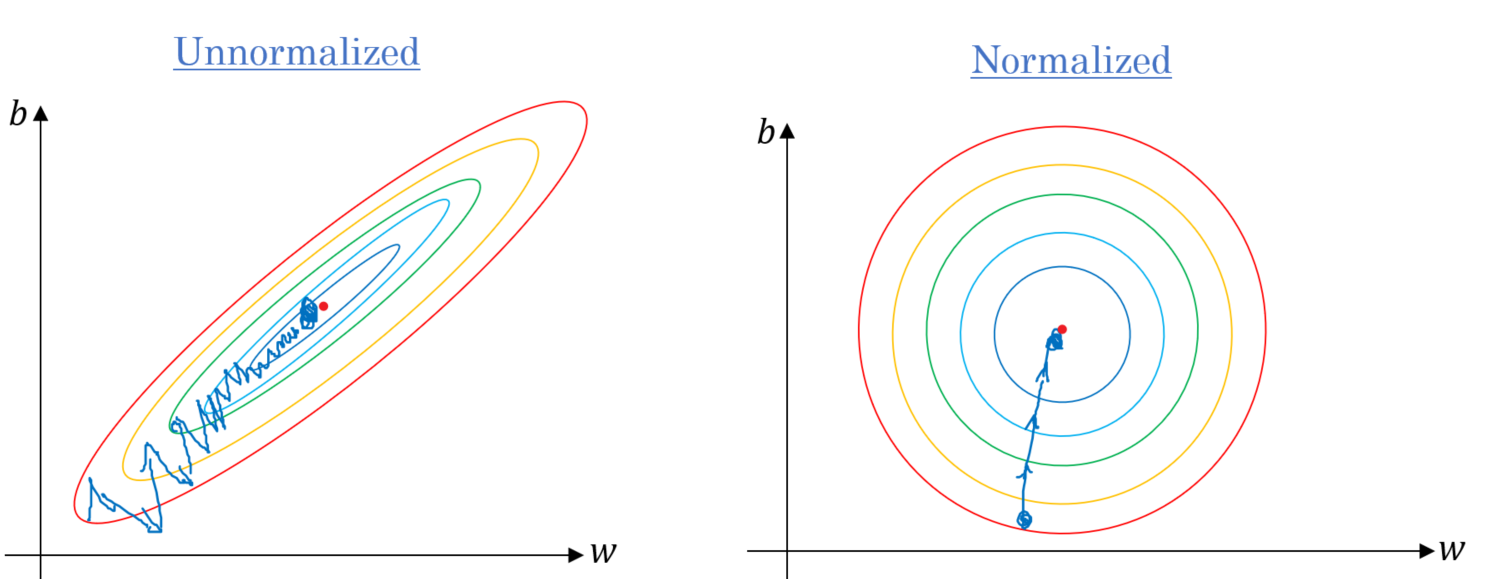Source: Towards Data Science

When using Gradient Descent, you should ensure that all features have a similar scale.

Cost function has the shape of a bowl. However, if the features have different scales, it will result in an elongated bowl.

For the Gradient Descent on a training set where features have the same scale, the algorithm goes straight toward the minimum, therefore reaching it quickly.

However, for the Gradient Descent on a training set where features does not have the same scale, the algorithm will first goes in a direction almost orthogonal to the direction of the minimum. It will then gradually march down an almost flat valley. It will take a long time before it reach the minimum.

### Types of Gradient Descent Algorithms

Batch Gradient Descent is a variation of the Gradient Descent algorithm that calculates the error for each example in the training dataset, but only updates the model after all training examples have been evaluated.

Since it uses the whole batch of training data to compute the gradient at every step, it result in an extremely slow computation.1593784740

## Introduction to Gradient Descent Algorithm

Imagine that you are standing at the top of a mountain,blindfolded. You are asked to move the down the mountain and find the valley. What would you do? Since you are unsure of where and in which direction you need to move to reach the the ground, you would probably take little baby steps in some direction of the higher incline and try to figure out if that path leads to your destination. You would repeat this process until you reach the ground. This is exactly how Gradient Descent algorithm works.

Gradient Descent is an optimisation algorithm which is widely used in machine learning problems to minimise the cost function. So wait! what exactly is cost function?

## Cost Function

Cost function is something which you want to minimise. For example, in case of linear regression, when you try to fit a line to your data-points, it may not fit exactly to each and every point in the data-set. Cost function helps us to measure how close the predicted values are to their corresponding real values. If x is the input variable and y is the output variable and h(hypothesis) is the predicted output of our learning algorithm.

hθ(x) = θ0 + θ1x , where θ0 is the intercept and θ1 is the gradient or slope.

Our aim here is to minimise the error between output y and the predicted output hθ(x). i.e. to minimise (hθ(x) — y)**2. This also called sum of the square error. Mathematically this cost function can be written as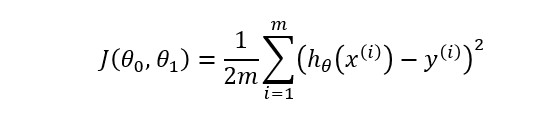Cost function of Linear Regression

Our aim here is to determine values for θ which make the hypothesis as accurate as possible. In other words, minimise J(θ0,θ1) as much as possible.

So now that we have some idea about the cost function, let us try to understand how exactly Gradient Descent helps us to reduce this cost function.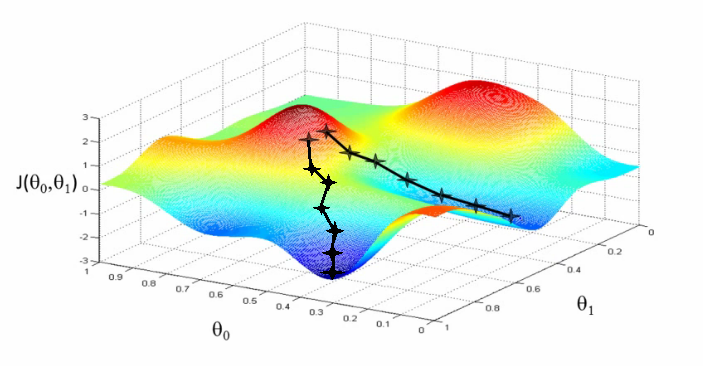Gradient Descent (Image Source : Coursera)

We run our algorithm with some initial weights (θ0 and θ1) and gradient descent keeps updating these weights until it finds the optimal value for these weights which result in minimising the cost function.

In simple terms our aim to move from the red region to blue region as shown in the above picture. Initially we start at any random values of θ0 and θ1 (say 0,0). In every step we keep updating the values of θ0 and θ1 by small amount to try and reduce J(θ0,θ1). We need to keep updating these values until we reach a local minimum.

It is also interesting to see that there can be multiple local minima’s for a given problem as seen in the above picture. Our starting point determines which local minima we end up on.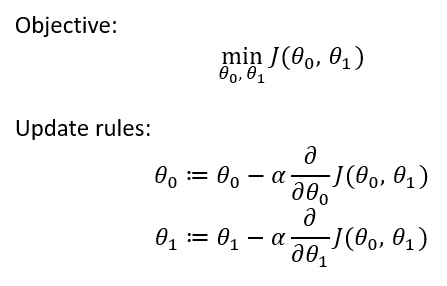Gradient Descent Objective & Update rules

Here we take partial derivative of cost function with respect to each of the weights. Here **alpha **symbol indicates Learning Rate.

Learning Rate

It is important to set learning rate at an optimal level. If the learning rate is too high then it results in too big steps which in-turn results in overshooting the minima and the gradient descent never converges to local minima. If the learning rate is too low, steps will be very small and gradient descent will take a lot of time to converge to local minima.

Another important thing to note is that we don’t have to change the value of alpha in each step. Since as the gradient decent approaches the global minimum, the derivative term gets smaller, so even the update gets smaller and algorithm takes smaller steps as it approach the minimum.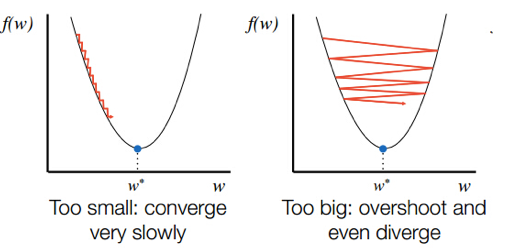Learning Rate (Image source : GitHub)

In case of Linear Regression cost function is always a **convex function (bowl shaped) **and always has a single minimum. So gradient descent will always converge to global optima.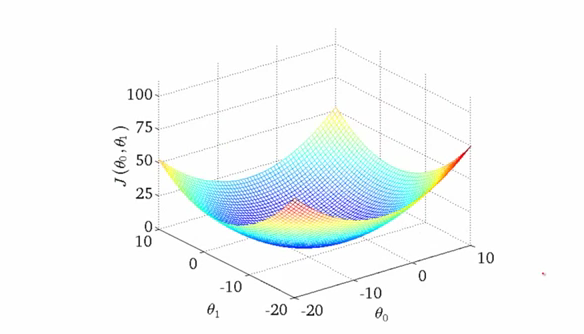Cost function of Linear Regression

## Conclusion

Gradient Descent helps us to reduce the cost function which results in increasing the accuracy of our machine learning model. Due to its ability to reduce error and since it can be applied to large data-sets with many variables, it is used in most of the machine learning algorithms.